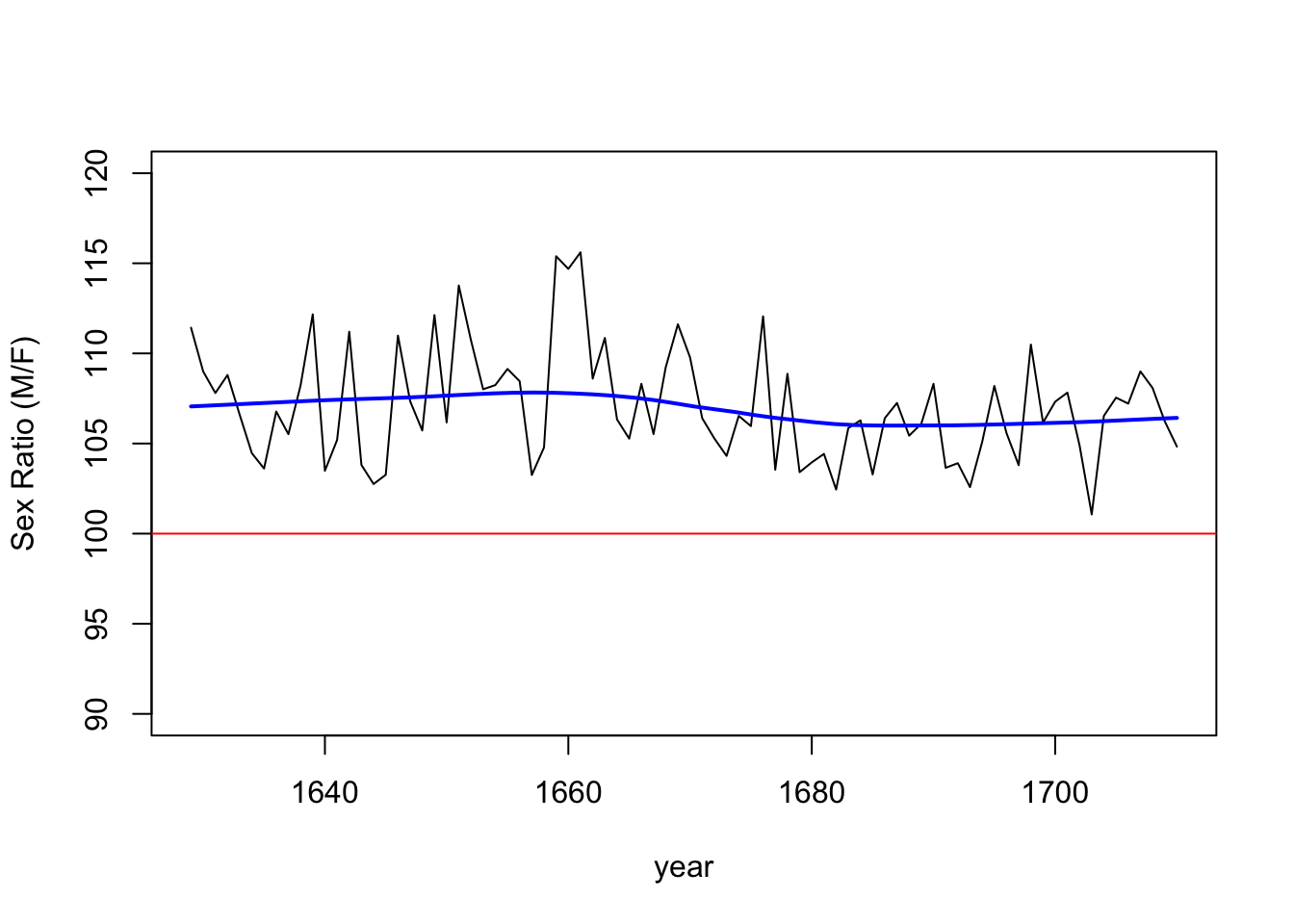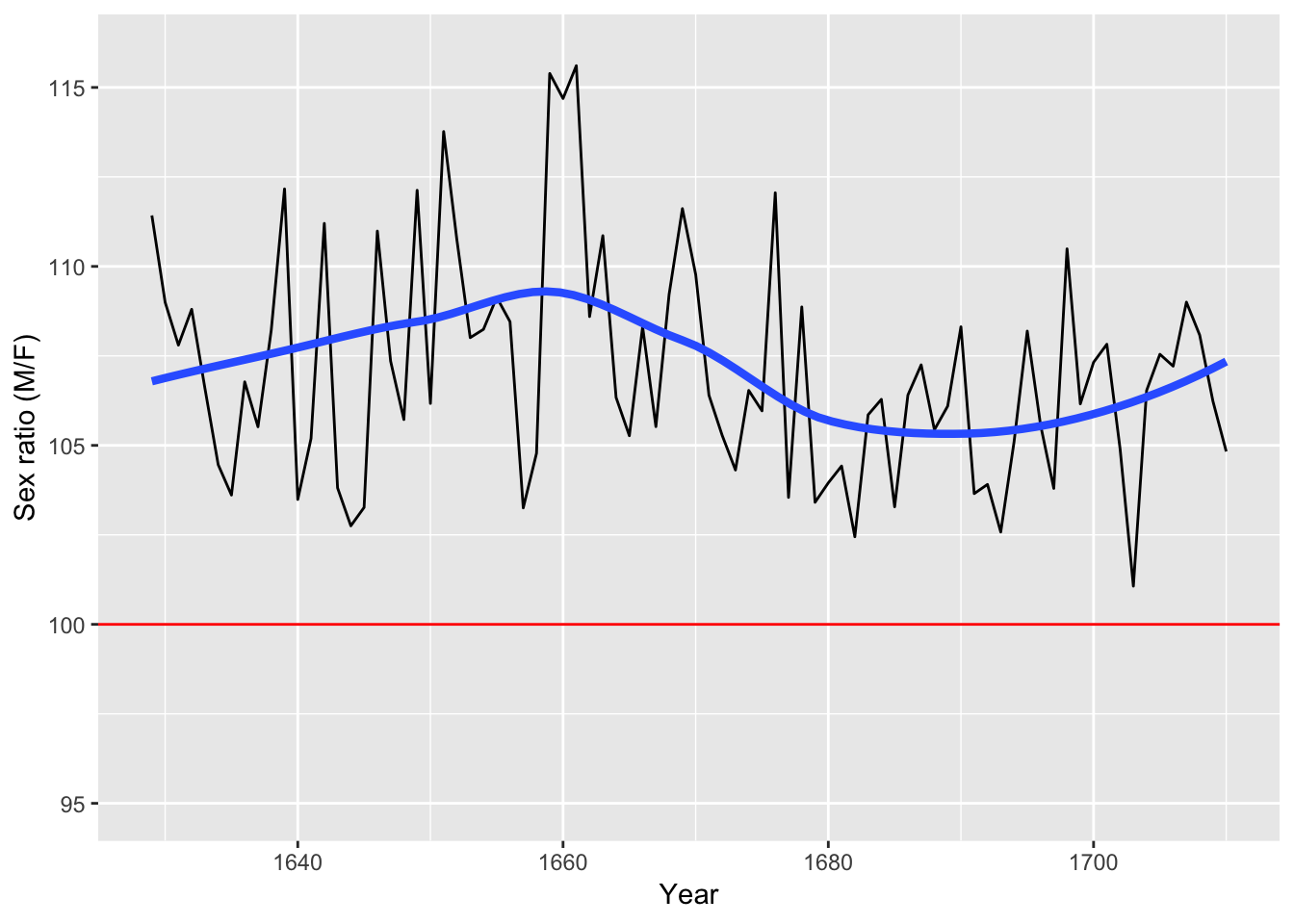Data is in 10-1-sex-ratio-data-x.csv, and can be downloaded from many sources- this version has only 3 variables and comes from OpenIntro.

library(ggplot2)
attach(Arbuthnot) #adds Arbuthnot to search path
ratio=100*boys/girls # define variable ratio
total=boys+girls # define variable total

First in R base graphics

plot(year,ratio, type='l', ylim=c(90, 120), ylab="Sex Ratio (M/F)")
abline(h=100, col="red")
Arb.smooth <- with(Arbuthnot, loess.smooth(year,ratio))
lines(Arb.smooth\$x, Arb.smooth\$y, col="blue", lwd=2)Figure 10.1 The sex ratio (number of boys per 100 girls) for London baptisms between 1629 and 1710, published by John Arbuthnot in 1710. The solid line represents an equal number of boys and girls; the curve is fitted to the empirical data. In all years there were more baptized boys than girls.

Then in qplot

q <- qplot(year,ratio, geom="line", ylim=c(95,116) ,xlab="Year", ylab="Sex ratio (M/F)") # constructs q initial plot object,
q <- q + geom_smooth(method = "loess", size = 1.5, se=FALSE) # add local regression for smoothness, as visual aid
q <- q + geom_hline(yintercept=100, col="red") # adds horizontal reference line at y=100
qThen in ggplot

p <- ggplot(Arbuthnot, aes(x=year, y=ratio)) # constructs initial plot object, p
p <- p + ylim(95,116) # adds y-limits
p <- p + geom_line(size=1.5) # connects the observations
p <- p + geom_smooth(method = "loess", size = 1.5, se=FALSE) # add local regression for smoothness, as visual aid
p <- p + geom_hline(yintercept=100, col="red") # adds horizontal reference line at y=100
p <- p + labs(x="Year", y="Sex ratio (M/F)") # Adds y-axis label and caption
p <- p + scale_colour_brewer(palette = "Accent") # sets the colour palette
p <- p  + scale_size_continuous(name = "Size", guide = FALSE)  # turns off otherwise added size legend
p <- p + theme(legend.position="none")#, legend.box = "horizontal") # removes the legend
p <- p + theme(plot.caption=element_text(hjust = 0.5)) # centre justifies the caption
p # displays the plot